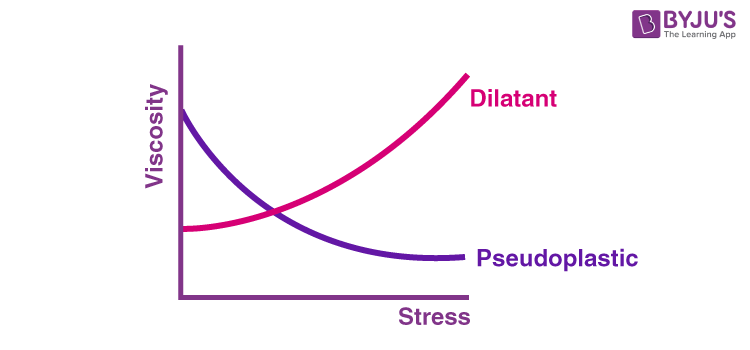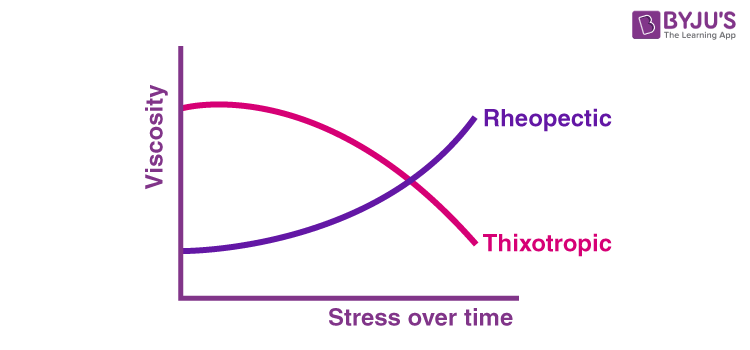# Newton’s Law of Viscosity

## Statement of Newton’s Law of Viscosity

According to Newton’s law of viscosity

The shear stress is directly proportional to the velocity gradient. The shear stress between the two adjacent layers of the fluid is directly proportional to the negative value of the velocity gradient between the same two adjacent layers of the fluid.

Mathematically, it is represented as:

 $$\begin{array}{l}\tau yx=-\mu (\frac{dVx}{dy})\end{array}$$

Where μ is the constant of proportionality known as dynamic viscosity whose unit id N.s.m-2.

## Types of Fluids

There are two types of fluids based on Newton’s law of viscosity:

• Newtonian fluids
• Non-Newtonian fluids

## Newtonian Fluids

The fluid whose viscosity remains constant is known as the Newtonian fluid. These fluids are independent of the amount of shear stress applied to them with respect to time. The relationship between the viscosity and shear stress of these fluids is linear.

• Water
• Alcohol
• Mineral oil
• Gasoline

## Non-Newtonian Fluids

The fluid whose viscosity changes when shear stress is applied is known as the Non-Newtonian fluids. These fluids are the opposite of Newtonian fluids.

• Toothpaste
• Ketchup
• Cosmetics
• Paint

### Types of Non-Newtonian Fluids

There are four types of Non-Newtonian fluids, and they are:

• Dilatant: The viscosity of these fluids increases when shear stress is applied. Quicksand, cornflour with water, and putty are examples of dilatant fluids.
• Pseudoplastic: The viscosity of these fluids decreases when shear stress is applied. These fluids are the opposite of dilatant fluids. Ketchup is an example of pseudoplastic.
• Rheopectic: The viscosity of these fluids increases when shear stress is applied along with time. They are similar to dilatant fluids, however, these fluids are time-dependent. Cream and gypsum paste are examples of rheopectic fluids.
• Thixotropic: The viscosity of these fluids decreases when shear stress is applied along with time. Cosmetics and paint are examples of thixotropic fluids.The other way of categorizing the Non-Newtonian fluids are based on their shear stress or the shear rate behaviour:

• The fluids whose shear stress is dependent on the time
• The fluids whose shear stress is independent of the time

## Frequently Asked Questions – FAQs

Q1

### What is the unit of consistency index K?

The unit of consistency index K is N.sn/m2.

Q2

### What happens to the shear stress in case of a rheopectic fluid?

In rheopectic fluid, the shear stress increases with time.

Q3

### Give the equation that is used for explaining the pseudoplastic and dilatant fluids

The following is the equation that is used for explaining the pseudoplastic and dilatant fluids:

$$\begin{array}{l}\tau =\mu (-\frac{dv}{dy})^{n}\end{array}$$

Q4

### Give the equation that represents a Bingham plastic fluid, if the apparent viscosity is given as μ.

The equation that represents a Bingham plastic fluid, if the apparent viscosity is given as μ is given as below:

$$\begin{array}{l}\tau =\mu (-\frac{dv}{dy})+\tau _{y}\end{array}$$

Q5

### What is the value of flow behaviour index n for a pseudoplastic fluid?

The value of flow behaviour index n for a pseudoplastic fluid is

## Watch the video and understand why water flows faster than honey!Test your Knowledge on Newton's Law of Viscosity